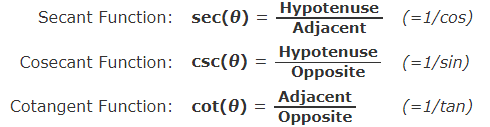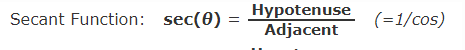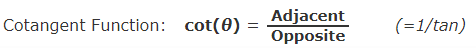# Find the trigonometric ratios indicated

#### jonah2.0

##### Full Member
Beer induced reaction follows.
Can someone please help solve this and show me how come about. This is an assignment but am yet to understand this topic: Thanks
That question is:
find the trigonometric ratios indicated

View attachment 29848
Looks pretty straightforward enough.
Just plug and chug.

#### Dr.Peterson

##### Elite Member
Can someone please help solve this and show me how come about. This is an assignment but am yet to understand this topic
I think you are saying that you have just begun studying trigonometric functions in a right triangle, and don't understand the definitions. In order to help, we need to see an attempt from you, either on part of this, or on a simpler example.

It may help if you understand that the answers will just be ratios of letters; for example, [imath]\sin(\phi)=\frac{a}{b}[/imath].

For the basics, see

#### ugudansam

##### New member
Yes am aware of
I think you are saying that you have just begun studying trigonometric functions in a right triangle, and don't understand the definitions. In order to help, we need to see an attempt from you, either on part of this, or on a simpler example.

It may help if you understand that the answers will just be ratios of letters; for example, [imath]\sin(\phi)=\frac{a}{b}[/imath].

For the basics, see
Yes am aware of such as sin(Θ)= a/b category and your reference link was actually perfect

#### Subhotosh Khan

##### Super Moderator
Staff member
Yes am aware of

Yes am aware of such as sin(Θ)= a/b category and your reference link was actually perfect
So you have completed this part of the assignment and you do not have any questions regarding this.

#### ugudansam

##### New member
So you have completed this part of the assignment and you do not have any questions regarding this.
no i don't, this diagram just seem complicated sir

#### Subhotosh Khan

##### Super Moderator
Staff member
no i don't, this diagram just seem complicated sir
Okay so you saw in the reference web-site

sin(Θ) = $$\displaystyle \frac{opposite}{hypotenuse}$$

In your assignment when you are

calculating sin(α)

opposite = ?​
hypotenuse = ?​

Staff member

#### ugudansam

##### New member
Okay so you saw in the reference web-site

sin(Θ) = $$\displaystyle \frac{opposite}{hypotenuse}$$

In your assignment when you are

calculating sin(α)

opposite = ?​
hypotenuse = ?​
i got stuck there as there are two switches

#### Subhotosh Khan

##### Super Moderator
Staff member
i got stuck there as there are two switches
Those are not "switches". I interpret those as two boxes. You should type in 107 in the top box and 277 in the bottom box

#### Subhotosh Khan

##### Super Moderator
Staff member
View attachment 29858
I do not understand the change here and e.g, sec(0) was suppose to be opposite/Hypotenuse and the (=1/Cos is another change)
You say

................ e.g, sec(Θ) was suppose to be opposite/Hypotenuse

Why do you think so?

You have

cos(Θ) = $$\displaystyle \frac{Adjacent}{Hypotenuse}$$....................... You learnt that from the referenced web-site. Now:

sec(Θ) = $$\displaystyle \frac{1}{cos(\theta)}$$ = $$\displaystyle \frac{Hypotenuse}{Adjacent}$$

correct?.................

#### ugudansam

##### New member
You say

................ e.g, sec(Θ) was suppose to be opposite/Hypotenuse

Why do you think so?

You have

cos(Θ) = $$\displaystyle \frac{Adjacent}{Hypotenuse}$$....................... You learnt that from the referenced web-site. Now:

sec(Θ) = $$\displaystyle \frac{1}{cos(\theta)}$$ = $$\displaystyle \frac{Hypotenuse}{Adjacent}$$

correct?.................

How is Sec related to Cos that Sec now have to be the inverse of Cos?

#### ugudansam

##### New member
You say

................ e.g, sec(Θ) was suppose to be opposite/Hypotenuse

Why do you think so?

You have

cos(Θ) = $$\displaystyle \frac{Adjacent}{Hypotenuse}$$....................... You learnt that from the referenced web-site. Now:

sec(Θ) = $$\displaystyle \frac{1}{cos(\theta)}$$ = $$\displaystyle \frac{Hypotenuse}{Adjacent}$$

correct?.................
as we have SOHCAHTOA formula for deriving the sin cos tan.
Is there any formula easy formula to use in deriving the likes of:Tutor have gotten to Trigonometric Ration of special angles. Everyone rushing the cos just to get out and not teaching students. Some move like jet and several Math class are thought at same hour hence the reason i find this here clearer than the tutors

#### Dr.Peterson

##### Elite Member
How is Sec related to Cos that Sec now have to be the inverse of Cos?

as we have SOHCAHTOA formula for deriving the sin cos tan.
Is there any formula easy formula to use in deriving the likes of:
View attachment 29861

Tutor have gotten to Trigonometric Ration of special angles. Everyone rushing the cos just to get out and not teaching students. Some move like jet and several Math class are thought at same hour hence the reason i find this here clearer than the tutors
It isn't clear what you are thinking.

Are you thinking that the secant (which doesn't have a "co") should be the reciprocal of the sine rather than of the cosine (which does have a "co")? If so, you are just imagining a pattern that doesn't exist. (You are not alone in that!)

They simply are defined as they are; for some reason, historically, what we call the secant was long ago thought of as more fundamental than what we call the cosine, so it was given a name of its own, while the cosecant was given a name derived from it.

This is a matter of definition, not derivation; no reason is needed for why something has the name it does.

If that is not what you were thinking, please explain better. Maybe, since you describe "SOHCAHTOA" as "deriving" the functions, what you really mean is, "Is there an easy way to memorize these definitions?" If so, then I just remember that the (non-co) secant is the reciprocal of the cosine, and the cosecant is the reciprocal of the (non-co) sine. The contrariness of the names is the mnemonic!

But in every case, the "co-function" is the function of the complement: coX(theta) = X(90 - theta). That is the part where the names are consistent.

Last edited:
•Subhotosh Khan

#### ugudansam

##### New member
What am deriving from your explanation is that:
Secant is not equals to SOH (Opposite/Adjacent) unlike Sine
Cosecant is not equal to CAH(Adjacent/Hypotenuse) unlike Cos. etc.
but let me assume that:Sec here does not look like sine in the inverse.

If you check the Tan category:#### Otis

##### Elite Member
may i confirm if i got the first row of my assignment correctly
Hi ugudansam. Yes, those are correct.

However, I think the adjacent side is labeled ω (lower-case Greek letter omega), instead of ε (lower-case Greek letter epsilon).

cos(θ) = ω/Z

sec(θ) = Z/ω

cot(θ) = ω/a#### ugudansam

##### New member
Hi ugudansam. Yes, those are correct.

However, I think the adjacent side is labeled ω (lower-case Greek letter omega), instead of ε (lower-case Greek letter epsilon).

cos(θ) = ω/Z

sec(θ) = Z/ω

cot(θ) = ω/aoh thank you but why am i not wrong with the sec and cot when i did not put --- Sec0 =z/w (=1/Cos0) ?

Last edited: